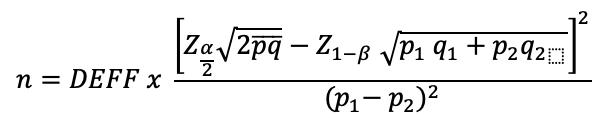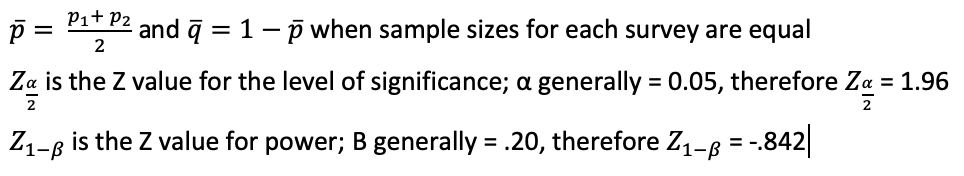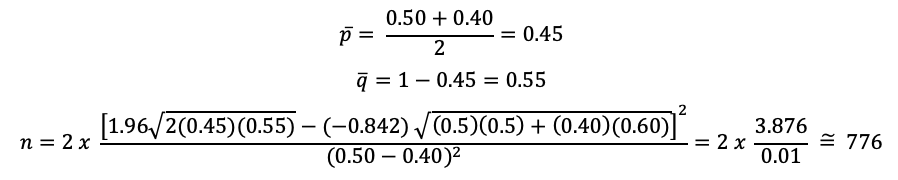Print View Documents

# Calculation of sample sizes for baseline and follow-up cluster surveys

Household surveys are frequently designed to estimate the prevalence of certain indicators and to assess changes in these indicators over time. Often, an initial survey serves as a baseline to identify the need for an intervention or to assess status before its implementation. A follow-up survey is then conducted to assess changes in selected indicators, and potentially to introduce additional indicators. The sample size for each survey should be estimated using survey design parameters that account for:

• assumptions about expected changes in the indicator estimates over a proposed time period and the reliability of the data to capture this change; and
• whether the same or different clusters and households will be included in the initial and follow-up surveys.
There are different methods to calculate the required sample size. One example is provided in the Feed the Future population-based survey sampling guide 1 and calculator2. You can also find details of the OpenEpi method at www.OpenEpi.com. To calculate the required sample size, the following estimates and assumptions are needed:

n is the calculated sample size.

DEFF is the estimated design effect (while the formula allows for one DEFF across the two surveys, it is recommended to use the larger DEFF for the sample size calculation)

Z is the statistic that defines the level of confidence required

α (“alpha”) is the desired level of two-sided significance of the difference in estimated proportions between surveys, usually 0.05 or 5% (corresponding to a 95% CI)

p is an estimate of the key indicator to be measured by the survey in the population group of interest, for example, the prevalence of iron deficiency among WRA, expressed as a proportion of that population

qi is 1 − pi

1 − β (the type II error) is the expected chance of detecting a difference between the two surveys, usually 0.8 (80%) or 0.9 (90%), also known as power

p1 is the estimate of the key indicator to be measured in the population group of interest, for example, prevalence of anaemia or proportion of households using adequately iodized salt at the time of the baseline survey

q1 is 1 − p1

p2 is the estimate of the key indicator to be measured in the population group of interest, expressed as a proportion, at the time of the follow-up survey

q2 is 1 − p2

In the formula below, it is assumed that the sample size in each of the two surveys will be the same. The formula is:Where:Table 5.1 and 5.2 display the different two-sided Z values (Zα/2) that can be used for different significance levels and the one-sided Z values (Z1-β) that can be used for various Power (1 − β) levels.

### Table 5.1 Two-sided Z values for different significance levels

Significance level (α) Two-sided Z value
0.01 2.576
0.05a 1.960
0.10 1.645

a Value used in example.

### Table 5.2 Two-sided Z values for different significance levels

β value Power (1 − β) One-sided Z value
0.01 .99 −2.326
0.05 .95 -1.645
0.10 .90 -1.282
0.20a .80 -0.842

a Value used in example.

An example of a calculation is shown in Box 5.6. It is important to remember that a higher power and lower significance level will increase the needed sample size

Once the baseline survey has been completed, the components assumed for the sample size calculation for the baseline (namely prevalence at baseline, DEFF, response rates and accuracy of projected estimates) for the follow-up survey should be revised based on the known information from the baseline survey.

You can find additional help in comparing the sample sizes of a baseline and a follow-up survey using the “Survey sample size calculator” online tool.

### Box 5.6 Example Sample Size Calculation for Baseline and Comparative Follow-Up Surveys

A country is going to begin fortifying flour with iron. The survey team estimates that the baseline prevalence of anaemia is 50% among WRA, and expects that iron fortification of flour will lower the anaemia prevalence in this group to 40% over 12 months.

Example of sample size calculation for those that wish to calculate this by hand:
p1 (proportion of anemia in the selected population group at baseline) = 0.50, q1 = 0.50
p2 (proportion of anemia in the selected population group at follow-up after intervention) = 0.40,
q2 = 0.60
α = 0.05, therefore Z(α/2) = 1.96
β = .20, therefore Z(1-β) = -.842
DEFF = 2

Assuming equal sample sizes, p is calculated as:In this example, the sample size would be 776 individuals in each cross-sectional survey, that is, 776 for the baseline survey and 776 in the follow-up survey. The number of households to visit to obtain information from 776 individuals would depend on the expected response rate and the proportion of the population group in each household.

1. Stukel DM. Feed the Future population-based survey sampling guide. Washington DC: Food and Nutrition Technical Assistance Project, FHI 360; 2018 (https://www.fantaproject.org/sites/default/files/resources/FTF-PBS-Sampling%20Guide-Apr2018.pdf, accessed 15 June 2020).

2. Population-based survey sample calculator (Excel file). Washington DC: Food and Nutrition Technical Assistance Project, FHI 360; 2018 (https://www.fantaproject.org/monitoring-and-evaluation/sampling, accessed 15 June 2020).

## Survey Sample Size Calculator

Sample size calculation spreadsheet for single and multiple cross sectional surveys